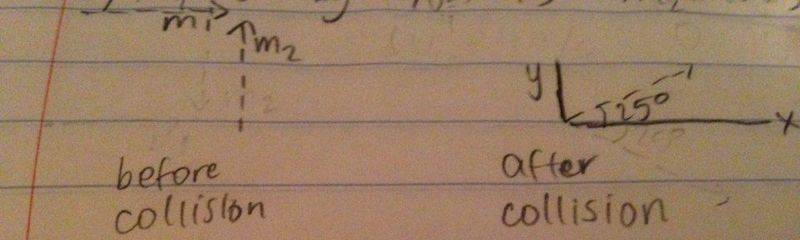# Ice skaters collision with angle

• fantisism

## Homework Statement

Two ice skaters crash into each other. Before they collide, one of them (50 kg) is skating in a straight line at 5 m/s, the other (40 kg) is skating at 4 m/s in a straight line at 90 degrees to the 50 kg skater’s direction. After the collision, the 50 kg skater is moving at 4 m/s at an angle of 25 degrees relative to their original direction. Calculate the velocity of the 40 kg skater after the collision. Is the total kinetic energy constant?

## Homework Equations

m1v1ix+m2v2ix=m1v1fx+m2v2fx
m1v1iy+m2v2iy=m1v1fy+m2v2fy

## The Attempt at a Solution

I drew a diagram before collision and after collision. Really, all I need to know is where in the formula(s) would I need to include the angle and I SHOULD be able to figure it out from there.You have v1fx and v1fy in the equations for components of momentum. How do you get them, knowing magnitude and angle of velocity ?

You have v1fx and v1fy in the equations for components of momentum. How do you get them, knowing magnitude and angle of velocity ?
Is v1fx=(4 m/s)(cos(25°)) and v1fy=(4 m/s)(sin(25°))?

Is v1fx=(4 m/s)(cos(25°)) and v1fy=(4 m/s)(sin(25°))?
Yes.

Yes.
And then with that information, solve for v2fx and v2fy?

And then with that information, solve for v2fx and v2fy?
Yes.

Yes.
And then once I figure out what those two velocities are, I would square them individually and take the square root of the sums? So, sqrt((v2fx)2+(v2fy)2? And that would be the answer for the first question?

And then once I figure out what those two velocities are, I would square them individually and take the square root of the sums? So, sqrt((v2fx)2+(v2fy)2? And that would be the answer for the first question?
The final velocity is asked. It is a vector with magnitude and direction. I think the problem wants both the magnitude and angle.

The final velocity is asked. It is a vector with magnitude and direction. I think the problem wants both the magnitude and angle.
To find the angle, would it be arctan(v2fy/v2fx)?

To find the angle, would it be arctan(v2fy/v2fx)?
Yes.

Alright. I can solve the second subquestion myself. Thank you again!